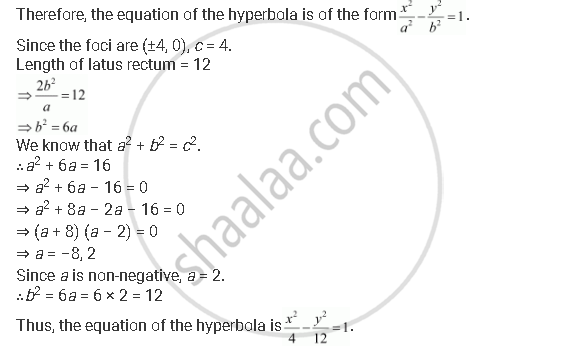CBSE (Arts) Class 11CBSE
Share
Notifications

View all notifications
Books Shortlist
Your shortlist is empty

# Find the Equation of the Hyperbola Satisfying the Give Conditions: Foci (±4, 0), the Latus Rectum is of Length 12 - CBSE (Arts) Class 11 - Mathematics

Login
Create free account

Forgot password?
ConceptHyperbola Latus Rectum

#### Question

Find the equation of the hyperbola satisfying the give conditions: Foci (±4, 0), the latus rectum is of length 12

#### Solution

Foci (±4, 0), the latus rectum is of length 12.

Here, the foci are on the x-axis.Is there an error in this question or solution?

#### APPEARS IN

Solution Find the Equation of the Hyperbola Satisfying the Give Conditions: Foci (±4, 0), the Latus Rectum is of Length 12 Concept: Hyperbola - Latus Rectum.
S